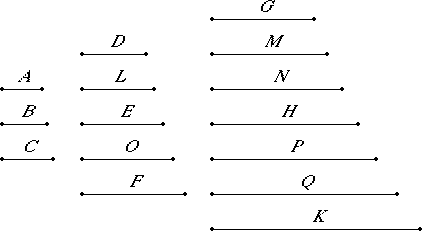# Proposition 13

If there are as many numbers as we please in continued proportion, and each multiplied by itself makes some number, then the products are proportional; and, if the original numbers multiplied by the products make certain numbers, then the latter are also proportional.

Let there be as many numbers as we please, A, B, and C, in continued proportion, so that A is to B as B is to C. Let A, B, and C multiplied by themselves make D, E, and F, and multiplied by D, E, and F let them make G, H, and K.

I say that D, E, and F and G, H, and K are in continued proportion.5

Multiply A by B to make L, and multiply the numbers A and B by L to make M and N respectively. Also multiply B by C to make O, and multiply the numbers B and C by O to make P and Q respectively.

Then, in manner similar to the foregoing, we can prove that D, L, and E and G, M, N, and H are continuously proportional in the ratio of A to B, and further E, O, and F and H, P, Q, and K are continuously proportional in the ratio of B to C.

VII.14

Now A is to B as B is to C, therefore D, L, and E are also in the same ratio with E, O, and F, and further G, M, N, and H in the same ratio with H, P, Q, and K. And the multitude of D, L, and E equals the multitude of E, O, and F and that of G, M, N, and H to that of H, P, Q, and K, therefore, ex aequali D is to E as E is to F, and G is to H as H is to K.

Therefore, if there are as many numbers as we please in continued proportion, and each multiplied by itself makes some number, then the products are proportional; and, if the original numbers multiplied by the products make certain numbers, then the latter are also proportional.

Q.E.D.

## Guide

The proposition says that if the terms of a continued proportion are squared or cubed, then the resulting sequences of numbers are also in continued proportion.

Suppose that the original continued proportion has three terms: a, b, c. Then form two more sequences

a2, ab, b2, bc, c2
and
a3, a2b, a b2, b3, b2c, b c2, c3

Each of these are in continued proportion with the same ratio as the original sequence. The alternate terms in the second sequence form the continued proportion of the squares of the original sequence where the ratio is duplicate of the original ratio. Likewise, every third term of the third sequence make up a continued proportion of the cubes of the original sequence where the ratio is triplicate of the original ratio.